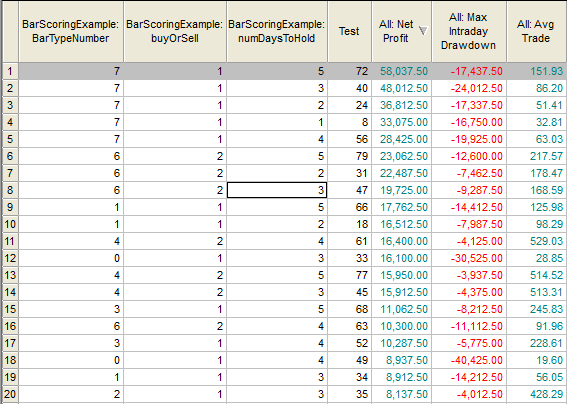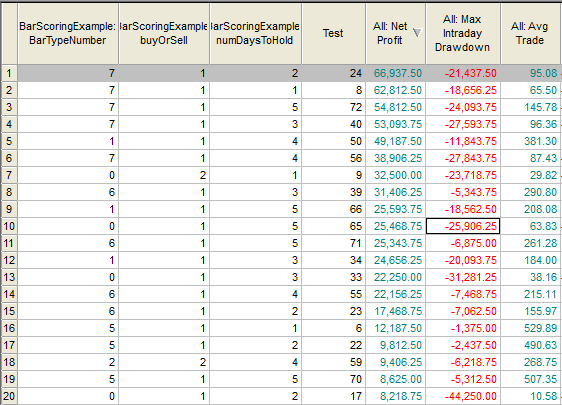# A Bar Scoring System – inspired by Keith Fitschen

In Keith’s wonderful book, “Building Reliable Trading Sytems”, he reveals several algorithms that classify an instruments’ movement potential.  In the part of the book that is titled Scoring by a Bar Type Criterion, he describes eight different two-day patterns that involve 3 different criteriaEight different Bar-Types

He looks at the relationship between today’s open and today’s close, today’s close and yesterday’s close, and today’s close in terms of the day’s range.  Bar-Types 1 to 4 all have the close of today >= close of yesterday.  Bar-Types 5 to 8 have close of today < close of yesterday.

I wanted to program this into my TradeStation and do some research to see if the concept is valid.  In his book, Keith tested a lot of different stocks and commodities.  In this post, I just test the ES, US, and Beans.  This form of research can be used to enhance an existing entry technique.

Here is how I defined the eight different bar types:

``````array : barTypeArray(false);

midRange = (h + l)/2;

barTypeArray = c >= c and c > o and c >= midRange;
barTypeArray = c >= c and c > o and c <  midRange;
barTypeArray = c >= c and c < o and c >= midRange;
barTypeArray = c >= c and c < o and c <  midRange;
barTypeArray = c <  c and c > o and c >= midRange;
barTypeArray = c <  c and c > o and c <  midRange;
barTypeArray = c <  c and c < o and c >= midRange;
barTypeArray = c <  c and c < o and c <= midRange;``````
Defining Eight Different Bar Types

I used a brute force approach by creating an 8-element array of boolean values.  Remember EasyLanguage uses a 0 index.  If the two -day pattern matches one of the eight criteria I assign the element a true value.  If it doesn’t match then a false value is assigned.  I use an input value to tell the computer which pattern I am looking for.  If I choose Bar-Type and there is a true value in that array element then I take a trade.   By providing this input I can optimize over all the different Bar-Types.

``````Input :
BarTypeNumber(0), // which bar type
numDaysToHold(2); //how many days to hold position

For cnt = 0 to 7 //remember to start at 0
Begin
If barTypeArray[cnt] = true then whichBarType = cnt;
end;

If whichBarType = BarTypeNumber then
begin
if buyOrSell = 2 then sellshort this bar on close;
end;``````
Loop Thru Array to find Bar Type

Here are some results of looping through all eight Bar-Types, Buy and Sell, and holding from 1 to 5 days.

ES – ten – year results – remember these are hypothetical results with no commission or slippage.Here’s what the equity curve looks like.   Wild swings lately!!Beans:BondsKeith was right – look at the Bar Category that bubbled to the top every time – the most counter-trend pattern.  My Bar-Type Number 7  is the same as Keith’s 8.  Here is the code in its entirety.

``````{Bar Scoring by Keith Fitschen
from his book "Building Reliable Trading Systems" 2013 Wiley}

Input : BarTypeNumber(0),
numDaysToHold(2);

vars: midRange(0);
array : barTypeArray(false);

midRange = (h + l)/2;

barTypeArray = c >= c and c > o and c >= midRange;
barTypeArray = c >= c and c > o and c <  midRange;
barTypeArray = c >= c and c < o and c >= midRange;
barTypeArray = c >= c and c < o and c <  midRange;
barTypeArray = c <  c and c > o and c >= midRange;
barTypeArray = c <  c and c > o and c <  midRange;
barTypeArray = c <  c and c < o and c >= midRange;
barTypeArray = c <  c and c < o and c <= midRange;

vars: whichBarType(0),cnt(0);

For cnt = 0 to 7
Begin
If barTypeArray[cnt] = true then whichBarType = cnt;
end;

If whichBarType = BarTypeNumber then
begin
if buyOrSell = 2 then sellshort this bar on close;
end;